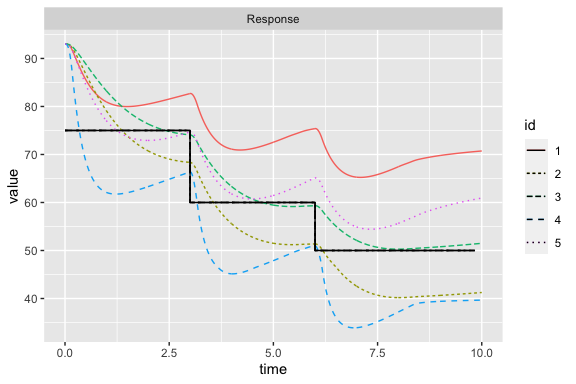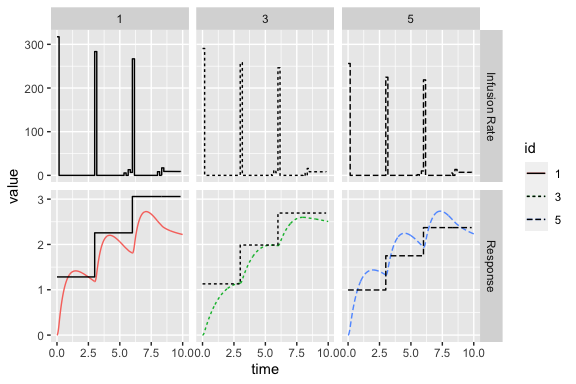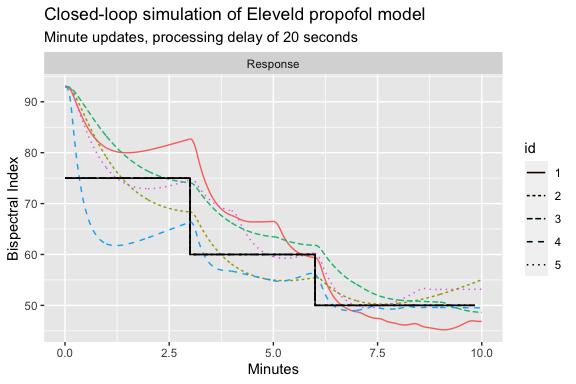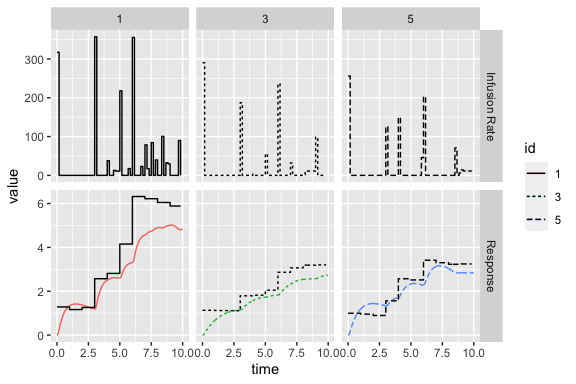# Population PK models

Population pharmacokinetic/pharmacodynamic (popPK) adjust model parameters (e.g., clearance) for patient covariate values (e.g., total body weight) that are believed to modify each parameter. By doing so, observable sources of variability between patients can be adjusted for, resulting in more personalized predictions.

PopPK models are implemented using the function poppkmod, which takes a data frame of patient covariate values. See ?poppkmod for appropriate covariate names for each model. The result is a poppkmod object that contains a list of the individual-level models (as pkmod objects), as well as information regarding the individual covariates and the model used. ID values are assigned to each individual (1 to N) if not supplied in the data argument.

In this example, we use the Eleveld popPK model for propofol (Eleveld et al. 2018), which describes the pharmacokinetics (PK) of propofol using a three-compartment effect site model. The effect site is linked to an Emax pharmacodynamic (PD) model that describes the Bispectral Index (BIS) response. BIS values are calculated from EEG sensor-derived data to measure a patient’s depth of anesthesia on a scale from 100 (fully awake) to 0 (fully anesthetized). BIS values are generated each second, but typically are smoothed in 10-15 second intervals, and are subject to a processing delay.

# create a data frame of patient covariates
data <- data.frame(ID = 1:5, AGE = seq(20,60,by=10),
TBW = seq(60,80,by=5), HGT = seq(150,190,by=10),
MALE = c(TRUE,TRUE,FALSE,FALSE,FALSE))
# create population PK model
pkpd_elvd <- poppkmod(data = data, drug = "ppf", model = "eleveld")

By default, poppkmod will calculate PK parameter values at the point estimates for each set of covariate values, that is, without inter- or intra-individual variability (IIV). Random sets of PK or PK-PD parameter values can be drawn from the IIV distribution either by setting sample=TRUE in poppkmod or by using the function sample_iiv. This can be useful when simulating responses under model misspecification. Parameters can be log-normally distributed (default, of the form $$\theta_i=\theta_{\text{pop}}e^{\eta_i}$$) or normally distributed $$\theta_i=\theta_{\text{pop}} + \eta_i$$) about the population parameter estimate. Random effects, $$\eta_i$$, are assumed to be normally distributed with variances given by the “Omega” matrix: $$\mathbf{\eta_i} \sim N(\mathbf{0},\mathbf{\Omega})$$.

set.seed(1)
pkpd_elvd_iiv <- sample_iiv(pkpd_elvd)

set.seed(1)
pkpd_elvd_iiv2 <- poppkmod(data = data, drug = "ppf", model = "eleveld", sample = TRUE)

identical(pkpd_elvd_iiv, pkpd_elvd_iiv2)
#>  TRUE

As with pkmod objects, the function inf_tci is used to calculate TCI infusion schedules. When supplied with a poppkmod, a separate infusion schedule is calculated for each individual and the results are returned in a single data frame with an ID variable.

target_vals = c(75,60,50,50)
target_tms = c(0,3,6,10)

# effect-site targeting
inf_poppk <- inf_tci(pkpd_elvd, target_vals, target_tms, "effect")
#>      id   begin     end inf_rate       Ct c1_start   c2_start    c3_start
#> [1,]  1 0.00000 0.16667 317.2861 1.283194 0.000000 0.00000000 0.000000000
#> [2,]  1 0.16667 0.33333   0.0000 1.283194 8.367531 0.05132774 0.003173343
#> [3,]  1 0.33333 0.50000   0.0000 1.283194 7.431692 0.14542679 0.009038266
#> [4,]  1 0.50000 0.66667   0.0000 1.283194 6.605881 0.22783591 0.014244659
#> [5,]  1 0.66667 0.83333   0.0000 1.283194 5.877130 0.29993684 0.018869910
#> [6,]  1 0.83333 1.00000   0.0000 1.283194 5.233999 0.36294839 0.022982287
#>       c4_start   c1_end     c2_end      c3_end    c4_end pdt pdresp_start
#> [1,] 0.0000000 8.367531 0.05132774 0.003173343 0.1069898  75     93.00000
#> [2,] 0.1069898 7.431692 0.14542679 0.009038266 0.3012965  75     92.42464
#> [3,] 0.3012965 6.605881 0.22783591 0.014244659 0.4687893  75     90.42123
#> [4,] 0.4687893 5.877130 0.29993684 0.018869910 0.6127194  75     88.18335
#> [5,] 0.6127194 5.233999 0.36294839 0.022982287 0.7359525  75     86.03425
#> [6,] 0.7359525 4.666396 0.41794570 0.026642014 0.8410145  75     84.08708
#>      pdresp_end
#> [1,]   92.42464
#> [2,]   90.42123
#> [3,]   88.18335
#> [4,]   86.03425
#> [5,]   84.08708
#> [6,]   82.37610

predict.poppkmod and simulate.poppkmod methods also can be used to predict and simulate values, respectively, using an infusion schedule.

predict(pkpd_elvd, inf = inf_poppk, tms = c(1,3))
#>       id time       c1        c2         c3        c4   pdresp
#>  [1,]  1    1 4.666495 0.4179535 0.02664251 0.8410303 82.37584
#>  [2,]  1    3 1.347430 0.7083844 0.04957118 1.2827736 75.00699
#>  [3,]  2    1 4.418211 0.3873929 0.02548454 0.7680677 82.74701
#>  [4,]  2    3 1.340578 0.6705145 0.04834295 1.2021802 75.03658
#>  [5,]  3    1 4.205641 0.3710587 0.02595592 0.7173458 82.81147
#>  [6,]  3    3 1.271022 0.6423528 0.04926224 1.1278927 75.04266
#>  [7,]  4    1 3.971108 0.3502109 0.02463783 0.6573044 83.12600
#>  [8,]  4    3 1.253788 0.6160778 0.04753211 1.0561426 75.09007
#>  [9,]  5    1 3.743670 0.3314773 0.02335556 0.6025752 83.42594
#> [10,]  5    3 1.232320 0.5918889 0.04576740 0.9882638 75.15224
set.seed(1)
simulate(pkpd_elvd_iiv, nsim = 3, inf = inf_poppk, tms = c(1,3), resp_bounds = c(0,100))
#>       id time     sim1     sim2     sim3
#>  [1,]  1    1 74.29153 72.07768 84.40919
#>  [2,]  1    3 84.63501 99.57543 74.00775
#>  [3,]  2    1 85.17350 86.28912 98.10793
#>  [4,]  2    3 77.67908 64.50018 73.27882
#>  [5,]  3    1 78.55654 91.56882 83.06531
#>  [6,]  3    3 57.58330 73.75215 81.12036
#>  [7,]  4    1 67.47856 68.12187 62.56494
#>  [8,]  4    3 70.27498 71.51372 53.27505
#>  [9,]  5    1 80.53896 76.06275 74.20240
#> [10,]  5    3 74.39156 66.22758 77.12749

While response values can be simulated using the simulate.poppkmod method, a more user-friendly function for conducting simulations is simulate_tci. simulate_tci can be used for pkmod or poppkmod objects and is used to both predict responses and simulate data values. Further, it easily incorporates model misspecification and can be used for closed-loop control if update times are specified (argument update_tms). At each update time, all “observed” (i.e., simulated) data up until the update time will be used to re-estimate model PK/PK-PD parameters using Bayes rule. When update times are used, simulate_tci can further incorporate processing delays so that simulated data will not be accessible to the update mechanism until the appropriate time (simulation time + processing time).

We first illustrate simulate_tci without updates and with observations generated every 10 seconds for 10 minutes. Infusions are calculated using the model parameters predicted at each patient’s covariates (object pkpd_elvd), while data values are simulated using model parameters sampled from the distribution of inter-individual variability (object pkpd_elvd_iiv). Data values are assumed to follow a normal distribution.

# values are in terms of minutes. 1/6 = 10 seconds
obs_tms <- seq(1/6,10,1/6)

sim_ol <- simulate_tci(pkmod_prior = pkpd_elvd, pkmod_true = pkpd_elvd_iiv,
target_vals, target_tms, obs_tms, type = "effect", seed = 1)

simulate_tci returns an object with class sim_tci that can be plotted using the ggplot2 library.

plot(sim_ol)Modifications can be made to the plot to show a subset of responses, concentrations instead of PD response values, infusion rates, and simulated data.

plot(sim_ol, yvar = "c4", id = c(1,3,5), show_inf = TRUE, wrap_id = TRUE)Closed-loop simulations can be implemented by specifying a set of update times. We illustrate this with updates each minute and a processing delay of 20 seconds.

sim_cl <- simulate_tci(pkmod_prior = pkpd_elvd, pkmod_true = pkpd_elvd_iiv,
target_vals, target_tms, obs_tms, update_tms = 1:10, delay = 1/3,
type = "effect", seed = 1)
#>  "Simulating ID=1"
#>  "Simulating ID=2"
#>  "Simulating ID=3"
#>  "Simulating ID=4"
#>  "Simulating ID=5"

Since plot.sim_tci returns a ggplot2 object, it is easy to modify aspects such as titles and axis labels using ggplot2 functions.

plot(sim_cl) +
xlab("Minutes") +
ylab("Bispectral Index") +
ggtitle("Closed-loop simulation of Eleveld propofol model",
subtitle = "Minute updates, processing delay of 20 seconds")plot(sim_cl, yvar = "c4", id = c(1,3,5), show_inf = TRUE, wrap_id = TRUE)